# Filtering a row in PySpark DataFrame based on matching values from a list

• Last Updated : 28 Jul, 2021

In this article, we are going to filter the rows in the dataframe based on matching values in the list by using isin in Pyspark dataframe

isin(): This is used to find the elements contains in a given dataframe, it will take the elements and get the elements to match to the data

Syntax: isin([element1,element2,.,element n])

Create Dataframe for demonstration:

## Python3

 `# importing module``import` `pyspark`` ` `# importing sparksession``from` `pyspark.sql ``import` `SparkSession`` ` `# creating sparksession``# and giving an app name``spark ``=` `SparkSession.builder.appName(``'sparkdf'``).getOrCreate()`` ` `# list  of students  data  with null values``# we can define null values with none``data ``=` `[[``1``, ``"sravan"``, ``"vignan"``],``        ``[``2``, ``"ramya"``, ``"vvit"``],``        ``[``3``, ``"rohith"``, ``"klu"``],``        ``[``4``, ``"sridevi"``, ``"vignan"``],``        ``[``5``, ``"gnanesh"``, ``"iit"``]]`` ` `# specify column names``columns ``=` `[``'ID'``, ``'NAME'``, ``'college'``]`` ` `# creating a dataframe from the lists of data``dataframe ``=` `spark.createDataFrame(data, columns)`` ` `dataframe.show()`

Output: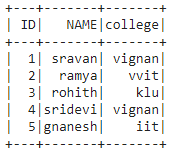## Method 1: Using filter() method

It is used to check the condition and give the results, Both are similar

Syntax: dataframe.filter(condition)

Where, condition is the dataframe condition.

Here we will use all the discussed methods.

Syntax: dataframe.filter((dataframe.column_name).isin([list_of_elements])).show()

where,

• column_name is the column
• elements are the values that are present in the column
• show() is used to show the resultant dataframe

Example 1: Get the particular ID’s with filter() clause.

## Python3

 `# get the ID : 1,2,3 from dataframe``dataframe.``filter``((dataframe.``ID``).isin([``1``,``2``,``3``])).show()`

Output: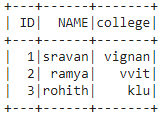Example 2: Get ID’s not present in 1 and 3

## Python3

 `# get the ID : not in 1 and 3 from dataframe``dataframe.``filter``(~(dataframe.``ID``).isin([``1``, ``3``])).show()`

Output: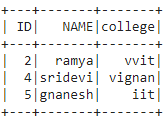Example 3: Get names from dataframe.

## Python3

 `# get name as sravan``dataframe.``filter``((``  ``dataframe.NAME).isin([``'sravan'``])).show()`

Output: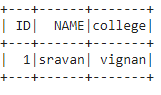## Method 2: Using where() method

where() is used to check the condition and give the results

Syntax: dataframe.where(condition)

where, condition is the dataframe condition

Overall Syntax with where clause:

dataframe.where((dataframe.column_name).isin([elements])).show()

where,

• column_name is the column
• elements are the values that are present in the column
• show() is used to show the resultant dataframe

Example: Get the particular colleges with where() clause

## Python3

 `# get college as vignan``dataframe.where((``  ``dataframe.college).isin([``'vignan'``])).show()`

Output: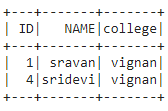My Personal Notes arrow_drop_up